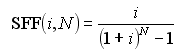# HOMER Pro 3.12

 Navigation: Glossary Sinking Fund FactorThe sinking fund factor is a ratio used to calculate the future value of a series of equal annual cash flows. The equation for the sinking fund factor is:where: i N = number of years

For example, for i = 7% and N = 5 years, the sinking fund factor is equal to 0.1739. Therefore, five annual payments of \$173.90 earning 7% interest are worth \$1000.00 at the end of the fifth year.

See also

Capital Recovery Factor